• 操作系统学习之用C语言模拟FIFO算法
2021-06-06 17:30:24

# 前言

FIFO算法比较简单，没什么好说的，就是先进先出。不过我添加了3状态，不过也只有堵塞，没有将阻塞进程唤醒的过程。

Buddy算法：操作系统学习之用C语言模拟伙伴(Buddy)算法
FIFO算法：操作系统学习之用C语言模拟FIFO算法
LRU算法：操作系统学习之用C语言模拟LRU算法
Clock算法：操作系统学习之用C语言模拟CLOCK算法
本源代码原创，转载请注明，同时由于本人才疏学浅，刚入门操作系统，如有错误敬请指教
本文原创，创作不易，转载请注明！！！
本文链接
个人博客：https://ronglin.fun/?p=199
PDF链接：见博客网站
CSDN: https://blog.csdn.net/RongLin02/article/details/117632249

# 算法模拟

## 算法解释

先来看看课本是怎么解释FIFO(First In First Out 先进先出)算法的。
FIFO策略把分配给进程的页框视为一个循环缓冲区，并按循环方式移动页。他需要的只是的一个指针，该指针在进程的页框中循环。因此这是一个实现起来最简单的页面置换策略。除简单外，这种方法所隐含的逻辑是置换驻留在内存中时间最长的页：很久以前换入内存的页，现在可能不会再用到。这一推断通常是错误的，因为经常会出现一部分程序或数据在整个程序的生命周期中使用频繁率都很高的情况，若使用FIFO算法，则这些页需要被反复地换入和换出。 ——操作系统-精髓与设计原理(第九版)P227

## 代码解释

简单的来说，就是这个算法实现起来很简单，但是由于程序局部性原理，可能会产生很高的缺页率，简单的用C模拟一下，如果内存调用进程，用FIFO算法，会大概发生什么情况。
来一个进程，先判断当前内存剩余大小能否放下，如果放不下，就搁置这个进程，如果放的下。就判断其是否需要io操作，如果需要，把状态切换到阻塞态,如果不需要就变成运行态。

## 源代码

直接看源代码叭：

#include<stdio.h>
#include<stdlib.h>
#include <time.h>
#define MAX_NUM_PROC 10
#define MAX_PROC_SIZE 300
#define MAX_MEMORY 1024

//进程结构体
//state 阻塞-1 就绪0 运行1
struct Process
{
int pid;
int ppid;
int state;
int p_size;
int io;

void init()
{
pid = -1;
ppid = -1;
state = 0;
p_size = 0;
io = 0;
}
}procs[MAX_NUM_PROC];

void printProc(struct Process proc);

int main()
{
int use_memory=0;
for(int i=0;i<MAX_NUM_PROC;i++)
{
procs[i].init();
procs[i].pid=i+1;
if((i+1)%3==0)
procs[i].io=i+1;
srand((unsigned)time(NULL)*i);
procs[i].p_size=rand() % MAX_PROC_SIZE +1;
}

for(int i=0;i<MAX_NUM_PROC;i++)
{
if(MAX_MEMORY - use_memory - procs[i].p_size > 0)
{
procs[i].state = 1;
use_memory += procs[i].p_size;
if(procs[i].io)
{
procs[i].state = -1;
}
}
else
{
procs[i].state = 0;
}

}

printf("%d\n",use_memory);
for(int i=0;i<MAX_NUM_PROC;i++)
{

printProc(procs[i]);
}

return 0;
}

void printProc(struct Process proc)
{
printf("pid = %d ",proc.pid);
printf("p_size = %d ",proc.p_size);
printf("io = %d ",proc.io);
printf("state = %d ",proc.state);
printf("\n");

}



## 输出解释

980
pid = 1 p_size = 39 io = 0 state = 1
pid = 2 p_size = 289 io = 0 state = 1
pid = 3 p_size = 171 io = 3 state = -1
pid = 4 p_size = 53 io = 0 state = 1
pid = 5 p_size = 235 io = 0 state = 1
pid = 6 p_size = 117 io = 6 state = -1
pid = 7 p_size = 299 io = 0 state = 0
pid = 8 p_size = 181 io = 0 state = 0
pid = 9 p_size = 63 io = 9 state = -1
pid = 10 p_size = 13 io = 0 state = 1


10个进程，第一行是使用的内存大小，p_size是进程所占用的空间，io是所需要的io请求序号，state是状态
一个进程刚开始被创建，如果内存放的下，就直接运行，状态变成1，如果有io请求，状态变成-1，如果内存放不下就保持0；

# 总结

本来最开始想用七状态模型写的，但是构思的时候发现很麻烦，要维护7个链表，时间关系，就简单的模拟一下叭 =w=操作系统
更多相关内容
• c 语言实现 fifo 算法及代码 C 语言是一门通用计算机编程语言应用广泛 C 语言的设计目标是提供一 种能以简易的方式编译 处理低级存储器产生少量的机器码以及不需要任何运行环境支 持便能运行的编程语言 尽管 C 语言...
• 实用文档 实 验 报 告 课程名称 学生所在系部 年级 专业班级 学生姓名 学号 任课教师 实验成绩 软件工程系制 实验题目 先进先出FIFO页面置换算法和最近最久未使用LRU置换算法程序设计 实验目的 通过对FIFOLRU算法的...
• FIFO先进先出算法实现：C语言中FIFO算法的实现
• c语言实现fifo算法及代码 在操作系统中，当程序在运行过程中，若其所要访问的页面不再内存中而需要把他们调入内存，但内存已无空闲空间时，为了保证该进程能正常运行，系统必须从内存调出一页程序或数据送磁盘的...

C语言是一门通用计算机编程语言，应用广泛。C语言的设计目标是提供一种能以简易的方式编译、处理低级存储器、产生少量的机器码以及不需要任何运行环境支持便能运行的编程语言。

尽管C语言提供了许多低级处理的功能，但仍然保持着良好跨平台的特性，以一个标准规格写出的C语言程序可在许多电脑平台上进行编译，甚至包含一些嵌入式处理器(单片机或称MCU)以及超级电脑等作业平台。

二十世纪八十年代，为了避免各开发厂商用的C语言语法产生差异，由美国国家标准局为C语言制定了一套完整的国际标准语法，称为ANSI C，作为C语言最初的标准。

First Input First Output的缩写，先入先出队列，这是一种传统的按序执行方法，先进入的指令先完成并引退，跟着才执行第二条指令。

FIFO(First Input First Output)，即先进先出队列。在超市购物之后会提着我们满满的购物车来到收银台排在结账队伍的最后，眼睁睁地看着前面的客户一个个离开。这就是一种先进先出机制，先排队的客户先行结账离开。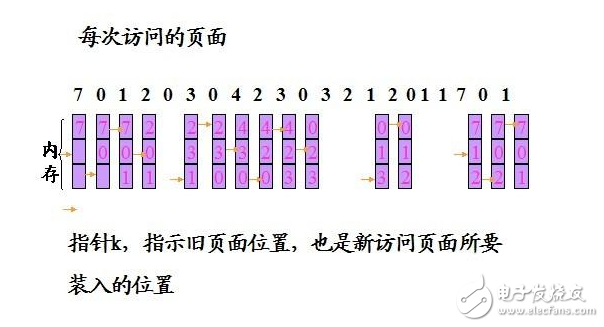c语言实现fifo算法及代码

在操作系统中，当程序在运行过程中，若其所要访问的页面不再内存中而需要把他们调入内存，但内存已无空闲空间时，为了保证该进程能正常运行，系统必须从内存调出一页程序或数据送磁盘的兑换区中。但哪一个页面调出，须根据一定的算法确定。通常，把选择换出页面的算法称为页面置换算法(Page-Replacement Algorithms)。置换算法的好坏将直接影响到系统的性能。

1) 先进先出(FIFO)页面置换算法

该算法总是淘汰最先进入内存的页面，即选择在内存中驻留时间最久的页面予以淘汰。该算法实现简单，只需把一个进程调入内存，按先后顺序排成一个队列，并设置一个指针，称为替换指针，使他总能指向最老的页面。但该算法与进程与实际运行的规律不相适应，效率最差。

2) 最近最久为使用(LRU)算法

LRU算法是根据页面调入内存后的使用情况进行决策的。就是利用“最近的过去”作为“最近的将来”的近似，因此是将最近最久未使用的页面予以淘汰。该算法赋予每一个页面一个访问字段，用来记录一个页面自上次被访问以来所经历的时间t，当淘汰一个页面时，选择现有页面中t值最大的，及最近最久为使用的页面予以淘汰。

167#include 《stdio.h》

#define PAGES 12 /*页面引用页数*/

#define M 3 /*当前分配给改作业的物理块数*/

//#define M 4

/*页面引用串*/

int page［PAGES］ = {4，3，2，1，4，3，5，4，3，2，1，5};

int rel［M］［PAGES］; /*存储结果数组*/

/*内存物理块结构体*/

typedef struct{

int pnum; /*该块中所存的页面号*/

int tm; /*从最近一次调入所经历的时间*/

}PBlock;

/*初始化物理块数组*/

void init(PBlock *pb)

{

int i，j;

//pb = (PBlock*)malloc(sizeof(PBlock)*M);

for(i=0;i《M;i++){

pb［i］.pnum = -1;

pb［i］.tm = -1;

for(j=0;j《PAGES;j++){

rel［i］［j］ = -1;

}

}

}

/*打印结果数组*/

void printRelArr(int rel［M］［PAGES］)

{

int i，j;

for(i=0;i《M;i++){

for(j=0;j《PAGES;j++){

if(rel［i］［j］==-1)

printf(“_ ”);

else

printf(“%d ”，rel［i］［j］);

}

printf(“\n”);

}

}

/*打印一维数组*/

void printArr1(int *arr，int n)

{

int i;

for(i=0;i《n;i++){

printf(“%d ”，arr［i］);

}

printf(“\n”);

}

/*查看页面号为num的页面是否在内存块中，存在返回1*/

int in_mem(int num，PBlock *pb，int m)

{

int i;

int b = 0;

for(i=0;i《m;i++){

if(pb［i］.pnum == num){

b = 1;

break;

}

}

return b;

}

/*FIFO 算法的实现，无需考虑时间*/

int fifo(PBlock *pb，int m)

{

int lps=0; /*缺页次数*/

double lpp; /*缺页率*/

int p = 0; /*替换指针*/

int index =0; /*页面号索引*/

while(index《PAGES){

if(！in_mem(page［index］，pb，M)){ //如果该页面不在物理块中

pb［p］.pnum = page［index］; /*将该页面放入物理块中*/

p = (p+1)%M; /*替换指针移动*/

lps++; /*却也次数加 1*/

for(int i=0;i《M;i++){

rel［i］［index］ = pb［i］.pnum;

}

}

index++;

}

printf(“FIFO算法所得缺页次数为 %d\n”，lps);

lpp = (double)lps/PAGES;

printf(“FIFO算法缺页率为 %0.4lf \n”，lpp);

printf(“页面号序列为：\n”);

printArr1(page，PAGES);

printf(“结果数列为：\n”);

printRelArr(rel);

return 0;

}

/*获得最近最久的块*/

int getP(PBlock *pb，int p)

{

int i;

bool out = true; //

for(i=0;i《M;i++){

if(pb［i］.tm == -1){

p = i;

out = false;

break;

}

}

if(out){

for(i=0;i《M;i++){

if(pb［i］.tm》pb［p］.tm)

p = i;

}

}

return p;

}

int getEQnum(int num，PBlock *pb)

{

int i;

int in = -1;

for(i=0;i《M;i++){

if(pb［i］.pnum == num){

in = i;

break;

}

}

return in;

}

/*LRU算法*/

void lru(PBlock *pb，int m)

{

int lps=0; /*缺页次数*/

double lpp; /*缺页率*/

int p = 0; /*替换指针*/

int index =0; /*页面号索引*/

while(index《PAGES){

if(！in_mem(page［index］，pb，m)){ /*如果页面不在物理块中*/

p = getP(pb，p);

pb［p］.pnum = page［index］;

pb［p］.tm = 0;

lps++;

for(int i=0;i《M;i++){

rel［i］［index］ = pb［i］.pnum;

}

}else{ /*如果页面在物理块中*/

int in = getEQnum(page［index］，pb); /*获取该页面在物理块中的索引*/

pb［in］.tm = 0;

}

int i;

for(i=0;i《M;i++){

if(i！=p&&pb［i］.tm！=-1){

pb［i］.tm++;

}

}

index++;

}

printf(“LRU算法所得缺页次数为 %d \n”，lps);

lpp = 1.0*lps/PAGES;

printf(“LRU算法缺页率为： %0.4lf\n”，lpp);

printf(“页面号序列为：\n”);

printArr1(page，PAGES);

printf(“LRU结果数组为：\n”);

printRelArr(rel);

}

int main()

{

//printArr(rel);

PBlock pb［M］;

init(pb);

fifo(pb，M);

init(pb);

lru(pb，M);

return 0;

}

FIFO的C语言实现

/*-----------------FIFO算法-------------------*/ /*算法描述：淘汰最先进入物理块的页面*/ /* ---------writen by Xu Zhuozhuo----------*/

C++代码示例：

#include 《iostream》

#define Num_Ref 20 //引用字符串的最大字符个数 using namespace std; int main() {

int num_ref; //用户的字符个数 int Phy_Blk［3］;

//物理块个数为3 int ref_arr［Num_Ref］;

//存放用户字符串

cout 《《“-----First In First Out-----” 《《endl 《《endl; do {

cout 《《“Please input the number of reference chars：” 《《endl; cin 》》num_ref;

//用户的字符个数 }while(num_ref》Num_Ref);

cout 《《“Please input the queue of reference chars：” 《《endl;

for(int i=0;i《num_ref;i++) //输入引用字符串

cin 》》ref_arr［i］;

for(int j=0;j《3;j++) //物理块数组初始化

Phy_Blk［j］=ref_arr［j］;

//FIFO，并输出本次替换结果

cout 《《endl 《《“Display the replacement procedure： ”;

cout 《《endl 《《“---------------------------------- ” 《《endl;

for(int loop=3;loop《num_ref;loop++)

{

Phy_Blk［0］=Phy_Blk［1］;

Phy_Blk［1］=Phy_Blk［2］;

Phy_Blk［2］=ref_arr［loop］;

cout 《《endl 《《“［” 《《loop-2 《《“］。 Physical block array content： ”;

for(int inloop=0;inloop《3;inloop++)

cout 《《Phy_Blk［inloop］ 《《“ ”;

}

return 0;

}

展开全文• TIA博途SCL语言_顺序队列FIFO算法_FB库文件
• TIA博途SCL语言_循环队列FIFO算法_FB库文件
• 操作系统FIFO算法C语言实现 前言 本机为微软Surface pro4，为64位，所用操作系统为Windos 10。本机虚拟机版本为Oracle VM VirtualBox 6.1.8，所用操作系统是使用Ubuntu18.04，。Ubuntu的虚拟硬盘设置为200G，显存为...

## 操作系统FIFO算法C语言实现

前言
本机为微软Surface pro4，为64位，所用操作系统为Windos 10。本机虚拟机版本为Oracle VM VirtualBox 6.1.8，所用操作系统是使用Ubuntu18.04，。Ubuntu的虚拟硬盘设置为200G，显存为128MB，内存为4GCPU2个，所用镜像源为清华大学软件镜像源。所使用linux内核为linux-5.11.8

注意事项
（1）本FIFO算法的实现思路是借鉴网上的相关资源，并非原创。
（2）本篇博客代码实现FIFO算法的命中率和置换显示。

FIFO算法介绍
FIFO算法：它的思想是先进先出（FIFO，队列），这是最简单、最公平的一种思想，即 如果一个数据是最先进入的，那么可以认为在将来它被访问的可能性很小。.空间满的时候，最先进入的数据会被最早置换（淘汰）掉 。

Linux下C语言实现FIFO算法的代码
1、使用文件编辑器在主目录下创建一个名为OS2的文件夹。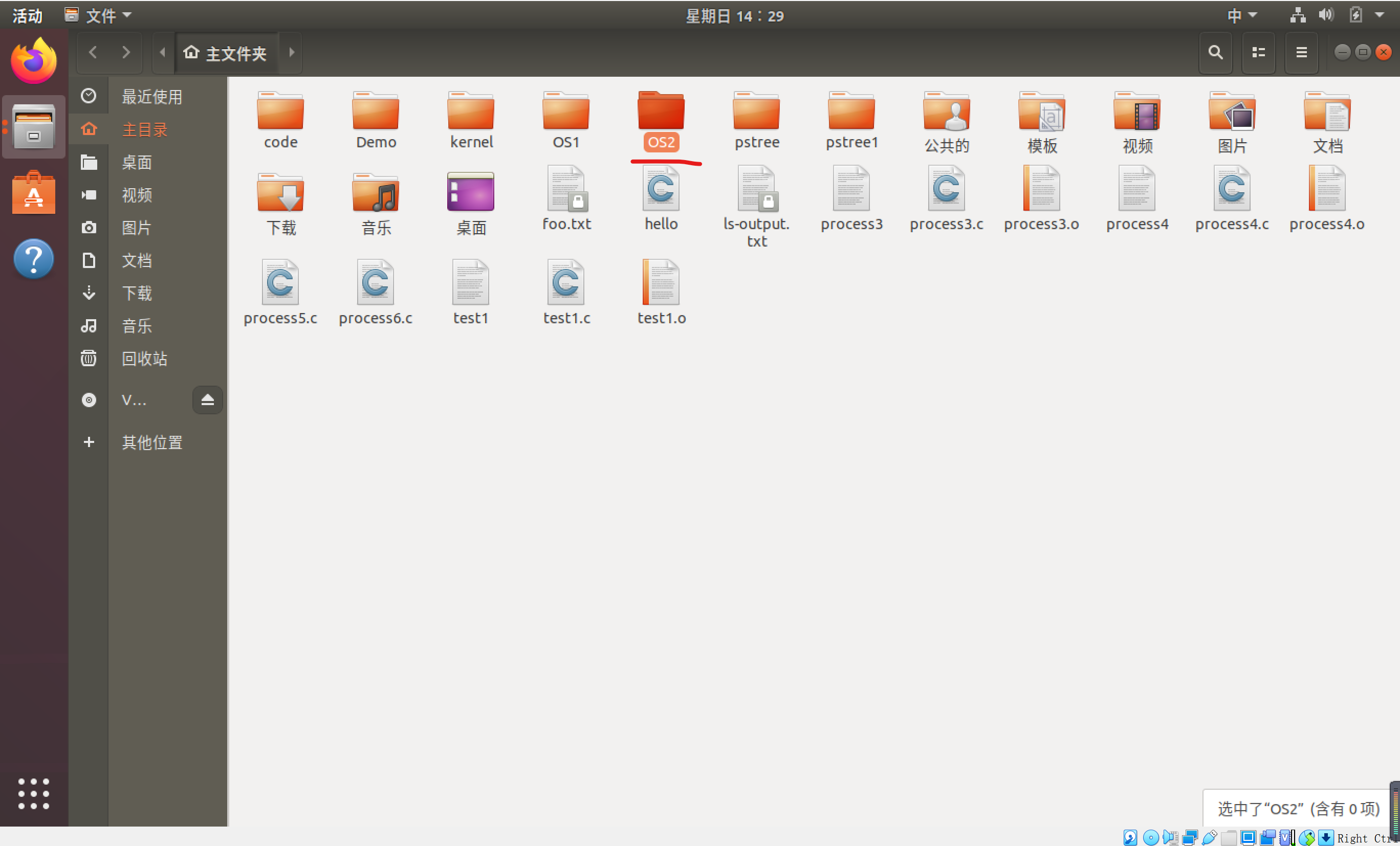2、打开Geany程序，然后点击新建按钮，创建一个新文件。
（注：创建完成界面如下）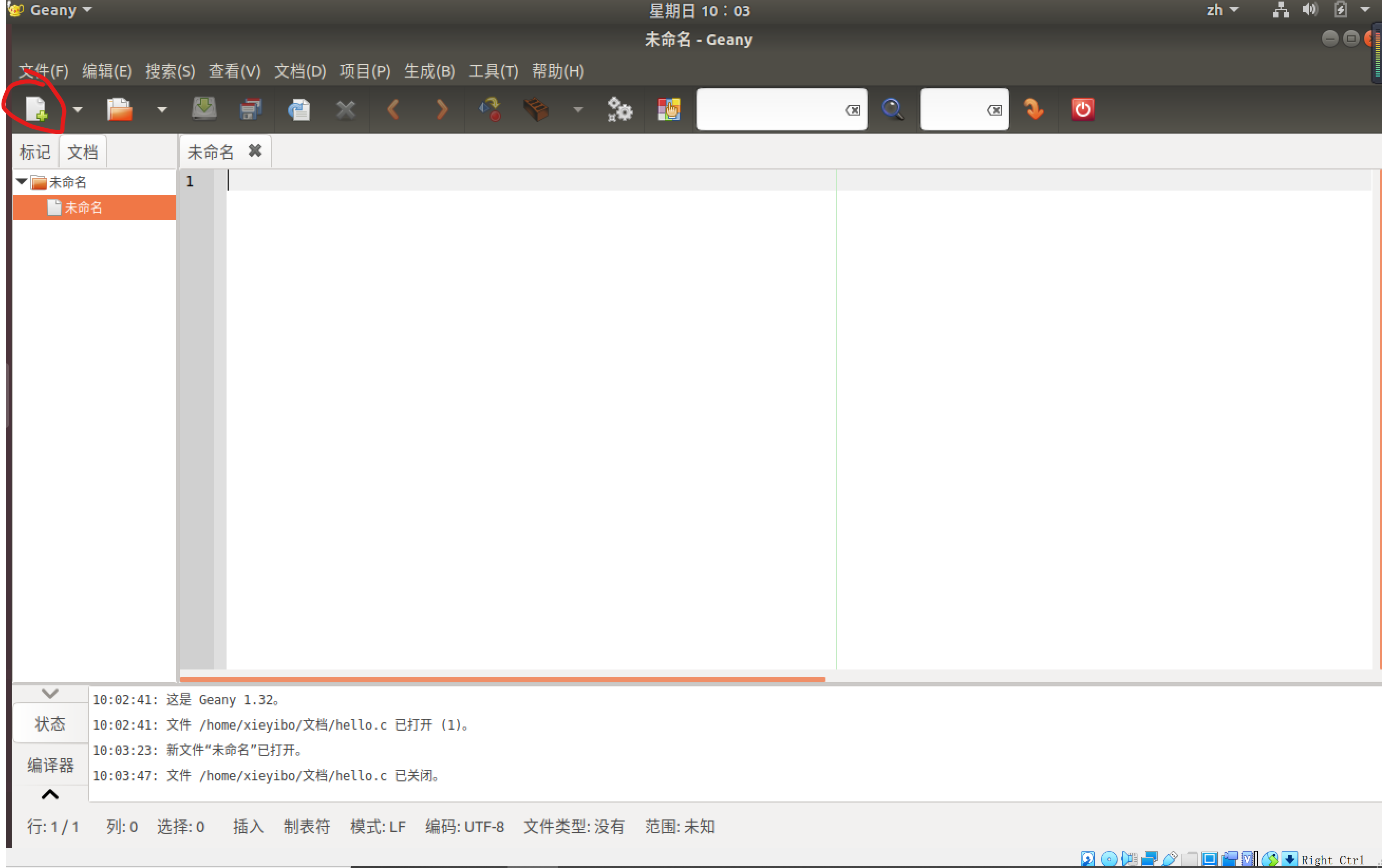3、在新建的文件中书写程序。（注：程序如下）

#include<stdio.h>
#include<stdlib.h>
#define N 320
int num[N];  //存放随机数
int page[N]; //存放页地址流
int mc; //memory capacity内存容量 ，并初始化为0

void randomnumber()//random number随机数 程序第一步，产生320个指令序列
{
int pc;
int flag=0;
scanf("%d",&pc);
printf("\n在0-319之间产生的320个随机数如下:\n");
for(int i=0;i<320;i++)
{
num[i]=pc;
if(flag%2==0) pc=++pc%320;  //flag=0||2 50%的指令是顺序执行的
if(flag==1) pc=rand()% (pc-1);   //flag=1 25%的指令是均匀分布在前地址部分
if(flag==3) pc=pc+1+(rand()%(320-(pc+1)));  //flag=3 25%的指令是均匀分布在后地址部分
flag=++flag%4;
printf("%3d   ",num[i]);
if((i+1)%10==0) printf("\n");  //每行输出10个数
}
}

{
for(int i=0;i<320;i++)
{
printf("%3d   ",page[i]=num[i]/10);
if((i+1)%10==0) printf("\n");  //每行输出10个数
}
}

//FIFO算法实现
int FIFO(int capacity)
{
int j,x,y,m;
int sum=0; //mc中分配的个数
int exist=0; //命中次数
int flag; //标志是否命中 flag=0没命中 flag=1命中
int ep=1; //elimination position淘汰位置
mc=page;
printf(" %2d加入  \t",page);
for(m=1;m<=capacity;m++)   //输出当前内存块的存储情况
printf("%2d  ",mc[m]);
printf("\n");
sum+=1;
for(j=1;j<320;j++)
{
flag=0;
for(y=1;y<=sum;y++)   //判断这个页地址流是否命中
if(mc[y]==page[j]) {
exist++;
flag=1;
printf(" %2d命中  \t",page[j]);
for(m=1;m<=capacity;m++)   //输出当前内存块的存储情况
printf("%2d  ",mc[m]);
printf("\n");
break;}
//没命中
if(flag==0)
{
if(sum<capacity)  //还有空块
{for(x=1;x<=capacity;x++) //查找内存块中第一个空块
if(mc[x]==-1) {
mc[x]=page[j];
sum++;
printf(" %2d加入  \t",page[j]);
for(m=1;m<=capacity;m++)    //输出当前内存块的存储情况
printf("%2d  ",mc[m]);
printf("\n");
break;}
}
else if(sum>=capacity)
{
int t=mc[ep];
mc[ep]=page[j];
printf(" %2d置换%2d\t",page[j],t);
for(m=1;m<=capacity;m++)   //输出当前内存块的存储情况
printf("%2d  ",mc[m]);
printf("\n");
ep+=1;
if(ep==capacity+1) ep=1;
}
}
}
printf("\nexist=%d\n命中率=%lf",exist,exist/320.0);
}
int main()
{
int capacity; //内存块数
printf("请输入第一条指令号(0~319):");
randomnumber();
printf("\n指令序列对应的页地址流:\n");
printf("\n\n\n\t\t先进先出算法(FIFO):\n\n");
printf("请输入内存块数（4-32）：");
scanf("%d",&capacity);
for(int i=1;i<=32;i++)  //给数组赋初值
mc[i]=-1;
FIFO(capacity);
return 0;
}



4、将程序文件命名fifo.c为保存到OS2文件下。（注：如图所示）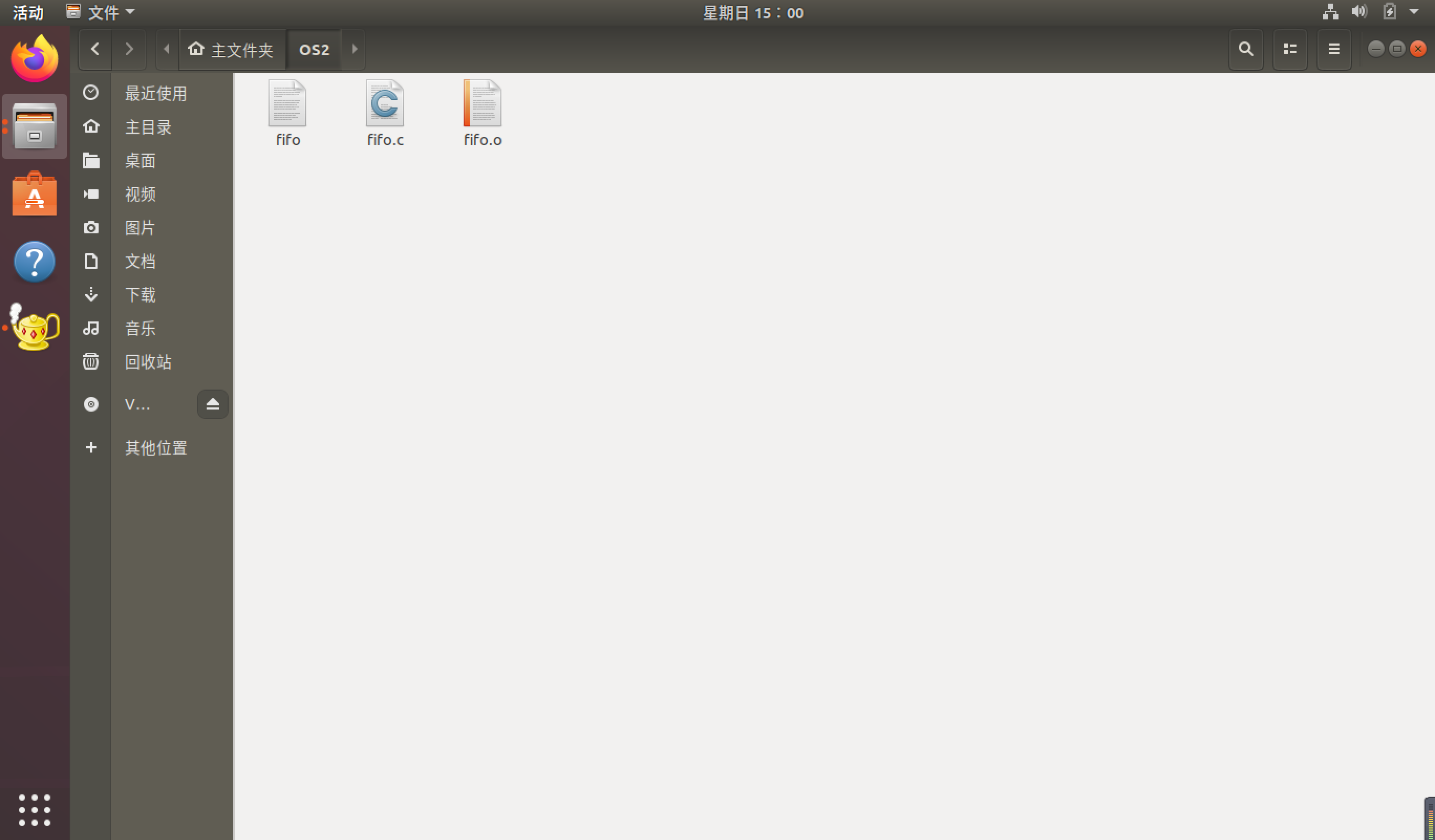FIFO算法实现效果如下
1、按如下图示中标明顺序点击按钮执行即可（注：效果图如下）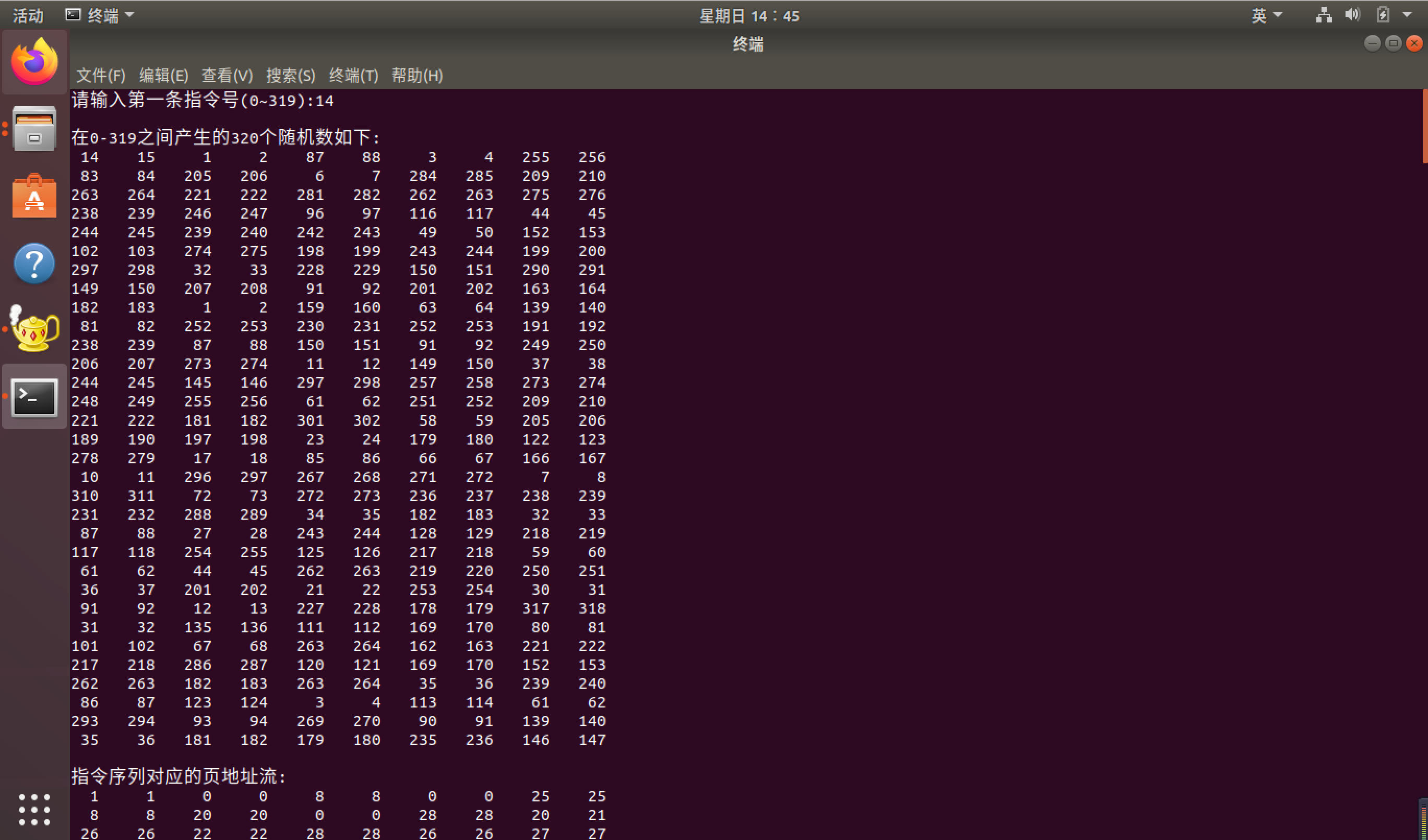展开全文• FIFO算法 FIFO算法是最简单的页面置换算法。FIFO算法为每个页面记录了调到内存的时间。当必须置换页面的时候，选择最旧的页面。 通俗来说，每次进入主存的时候 如果主存中不存在这个页面，那么最先进入的页面出队...

## FIFO算法

FIFO算法是最简单的页面置换算法。FIFO算法为每个页面记录了调到内存的时间。当必须置换页面的时候，选择最旧的页面。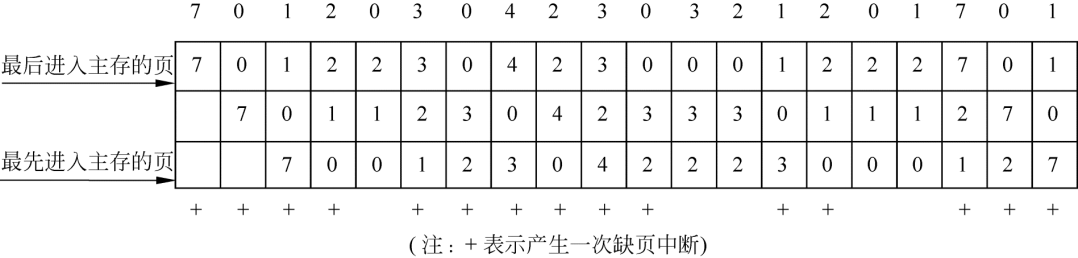通俗来说，每次进入主存的时候

• 如果主存中不存在这个页面，那么最先进入的页面出队。
• 如果主存存在这个页面，那么内存不动。

下面是C++面向对象写法代码。
这个说明一下为什么不用容器Queue。是因为queue并没有迭代器，所以无法去寻找里面是否含有某块页面。
直接使用线性表即可，方便简单且快速。

// 在分页式虚拟存储管理中，要求通过键盘输入分配给一个作业的物理块数和作业依次访问的10个页面号，
// 采用先进先出（FIFO）页面置换后
// ，顺序输出缺页中断时所淘汰的页面号，并计算缺页中断率。
#include <iostream>

using namespace std;
#define MAX 20
class Work
{
public:
void seta()
{
int c, i = 1;
cout << "输入10个页面号,以任意符号结尾" << endl;
cin >> c;
a = c;
while (cin >> c)
{

a[i] = c;
i++;
}
}
void geta()
{
cout << "10个页面号分别为:  ";
for (int i = 0; i < 10; i++)
{
cout << a[i] << " ";
}
cout << endl;
}
int index(int i)
{
return a[i];
}
~Work(){
cout<<"work 已被释放"<<endl;
}
private:
int a;
};

class space
{
public:
// 初始化构造函数，把除了物理块大小的其他空间都设置成其他值
// 将初始化空间设置成-1
space(int i)
{
size=i;
for (int j = 0; j < i; j++)
{
s[j] = -1;
}
s[i] = -999;
}

// 显示物理块现在的状态
void getSpace(){
int i=0;
cout<<"-------------物理块现在的状态是-------------"<<endl;

while(s[i]!=-999){
if(s[i]==-1){
cout<<"NaN"<<"     -||-      ";
i++;
continue;
}
cout<<s[i]<<"     -||-      ";
i++;
}
cout<<endl;
cout<<"------------------------------------------"<<endl;
}
int find(int n){
for(int i=0;i<size;i++){
if(s[i]==n){
return i;
}
}
return -1;
}

// 先进先出，去掉第一个物理块，改变最后一个物理块。
void change(int n){
for(int i=0;i<size;i++){
s[i]=s[i+1];
}
s[size-1]=n;

}

~space(){
cout<<"space 已被释放"<<endl;
}
private:
int s[MAX];
int size;
};

void FIFO(Work &a,space &s){
int i=0;
int local;
int count=0;
while(i<10){
if(s.find(a.index(i))==-1){
// 如果在物理块里面找不到作业
s.change(a.index(i));
// 那么就将物理块改变
s.getSpace();
count+=1;
}else{
s.getSpace();
}
i++;
}
double q;
q=(double)count/10;
cout<<"----------------------------------"<<endl;
cout<<"缺页中断的次数为： "<<count<<endl;
cout<<"缺页终端率为： "<<q<<endl;
cout<<"----------------------------------"<<endl;

}

int main()
{
Work a;
int num=0;
cout<<"输入物理块数的个数"<<endl;
cin>>num;
space s(num);
a.seta();
a.geta();
FIFO(a,s);

return 0;
}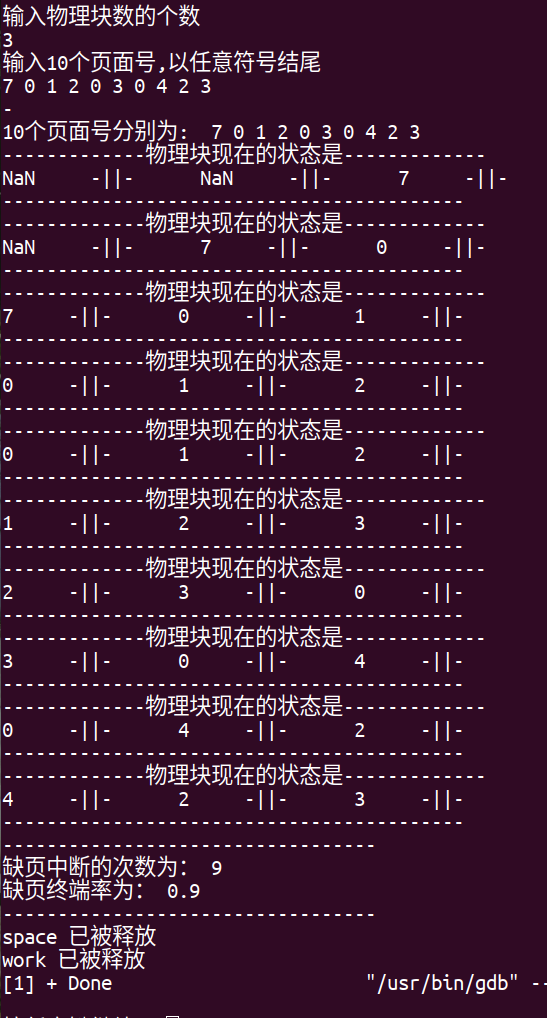展开全文c++
• 最简单的页面置换算法是先入先出（FIFO）法。这种算法的实质是，总是选择在主存中停留时间最长（即最老）的一页置换，即先进入内存的页，先退出内存。理由是：最早调入内存的页，其不再被使用的可能性比刚调入内存的...
• FIFO算法 文章目录FIFO算法概念一、解析二、代码实现 概念 FIFO（First Input First Output），即先进先出队列。可以类比 我们在饭堂排队打饭，先排到队伍的最后，等待前面的人一个个打完饭再轮到下一个。这就是一...数据结构
• 先进先出置换算法FIFO） 最简单的页面置换算法，淘汰最先调入的。 实现：队列 依据: 先进入的可能已经使用完毕。 基本思想： 当需要淘汰一个页面时，总是选择驻留主存时间最长的页面进行淘汰，即先进入主存的页面...操作系统 页面置换
• FIFO算法FIFO算法FIFO算法FIFO算法FIFO算法
• 话不多说直接放代码吧………… #include <iostream> #include <cstring> using namespace std; void dayinwulikuai(int *wulikuai,int wulikuaicnt) { int j; for(j=0;j<wulikuaicnt;..."bloc++ 操作系统
• 当从辅存调页至主存时，若主存已满时，需要进行主存页面之间的替换，虚拟存储器的替换算法有：FIFO算法、LRU算法、LFU算法等。 FIFO算法： 先进先出调度算法。如果一个数据是最先进入的，那么可能认为它被访问的可能...lru
• LRU、LFU、FIFO、Two queues（2Q）算法原理缓存 java
•操作系统 数据结构 java
• FIFO算法 #include #include #include #include #include #include #include #include #include #include using namespace std; int cnt; ///发生缺页的次数 const int maxn = 4; ///内存块数的数目 const int maxn...
• 操作系统存储管理中LRU算法和FIFO算法的模拟实现 使用纯C语言实现 在Linux下使用GNU编译器编译通过
• 作者 ：PoisonApple 转自http://www.elecfans.com/emb/604190.htmlFirst Input First Output...　FIFO（First Input First Output），即先进先出队列。在超市购物之后会提着我们满满的购物车来到收银台排在结账队伍...
• fifo算法实现系统的内存调度，平台VS6.0python 链表
• FIFO算法的c语言实现 详细代码 以及详细注释
• ## 【操作系统】FIFO算法

万次阅读 多人点赞 2019-07-27 14:13:27
A、考虑下述页面走向:6，7，5，2，6，7，3，6，7，5，2，3 当分配的内存物理块数量分别为3和4时: FIFO(先进先出页面置换算法)的缺页次数分别是多少? FIFO（先进先出页面置换算法）的缺页次数： FIFO（先进先出...
• 操作系统之存储管理——FIFO算法和LRU算法 操作系统之磁盘调度——SCAN实例讲解 一、实验目的 存储管理的主要功能之一是合理地分配空间。请求页式管理是一种常用的虚拟存储管理技术。 本实验的目的是通过请求...lru 操作系统...